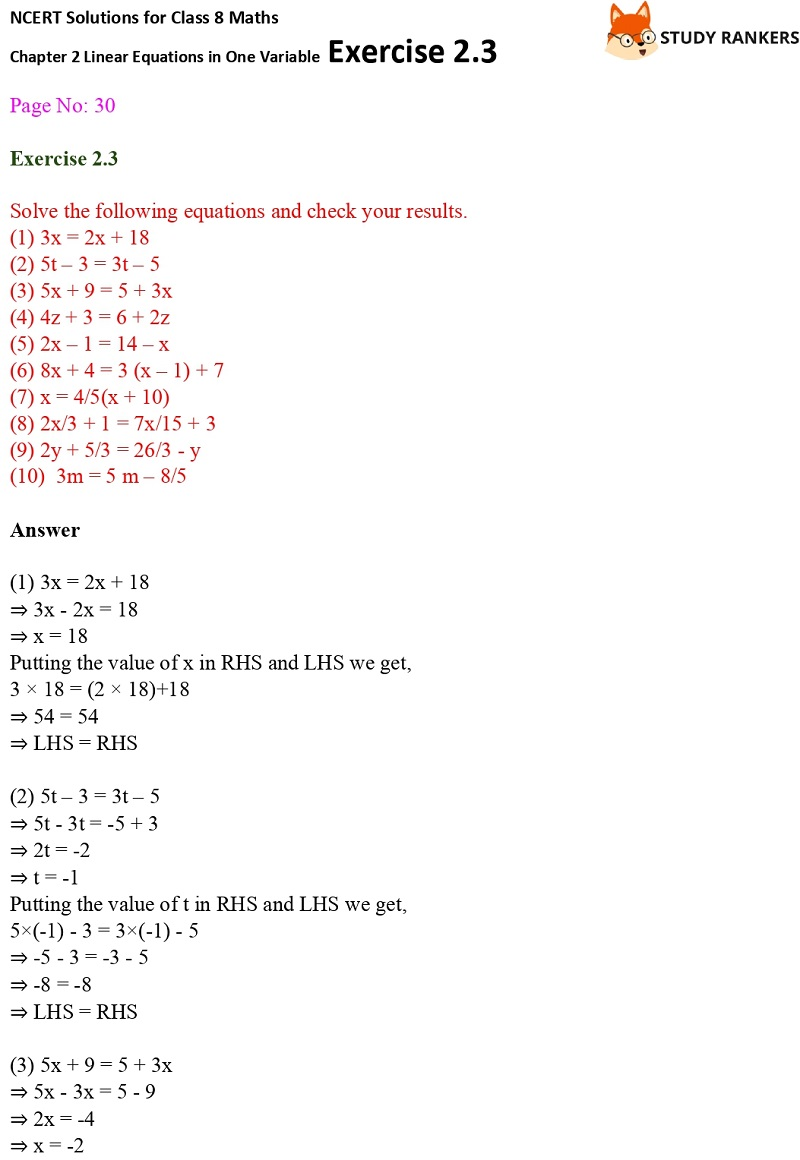>

# NCERT Solutions for Class 8 Maths Ch 2 Linear Equations in One Variable Exercise 2.3

NCERT Solutions for Class 8 Maths Ch 2 Linear Equations in One Variable Exercise 2.3 are given here which will be helpful for every student in clearing their doubts. These NCERT Solutions are prepared by Studyrankers experts which are detailed and accurate.

Also, NCERT Solutions for Class 8 Maths can be very helpful in inculcating correct learning habits among students. In exercise 2.3, you need to solve the equations having the variable on both side.X